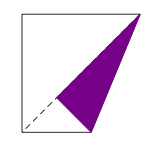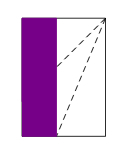# Origami Silver Rectangle

Alignments to Content Standards: 8.G.A.1 G-CO.D.12

This task examines the mathematics behind an origami construction of a rectangle whose sides have the ratio $(\sqrt{2}:1)$. Such a rectangle is called a silver rectangle.

Beginning with a square piece of paper, first fold and unfold it leaving the diagonal crease as shown here:Next fold the bottom right corner up to the diagonal:After unfolding then fold the left hand side of the rectangle over to the crease from the previous fold:Here is a picture, after the last step has been unfolded, with all folds shown and some important points marked. In the picture $T$ is the reflection of $S$ about $\ell$.1. Suppose $s$ is the side length of our square. Show that $|PT| = s$.
2. Show that $\triangle PQT$ is a 45-45-90 isosceles triangle.
3. Calculate $|PQ|$ and conclude that $PQRS$ is a silver rectangle.

## IM Commentary

The purpose of this task is to apply geometry in order analyze the shape of a rectangle obtained by folding paper. The central geometric ideas involved are reflections (used to model the paper folds), analysis of angles in triangles, and the Pythagorean Theorem. The task is appropriate either at the 8th grade level or in high school: the only difference would be the level of rigor expected in the explanation. The solution given is appropriate for either level.

The silver rectangle is one of three rectangles identified in ancient times as having important properties: the bronze rectangle has a side ratio of $(\sqrt{3},1)$ and the golden rectangle has a side ratio of $\left(1+\sqrt{5}:2 \right)$. Each of these three rectangles can be constructed by folding paper. Further properties of silver rectangles are examined in www.illustrativemathematics.org/illustrations/1489. Much more interesting information about the silver rectangle can be found here: http://en.wikipedia.org/wiki/Silver_ratio

This task could be given in a much more open ended form, particularly if done in high school. Teachers could go through the different steps of the construction and then prompt students to examine the ratio of side lengths in the final rectangle. There are many possible solution paths revolving around the different triangles in the picture that are similar: half of the original square, $\triangle TQP$, and the small triangle in the lower left of the last picture. These are all 45-45-90 triangles and the dimensions of the rectangle can be found by calculating the scale factor between any pair of these triangles.

The Standards for Mathematical Practice focus on the nature of the learning experiences by attending to the thinking processes and habits of mind that students need to develop in order to attain a deep and flexible understanding of mathematics. Certain tasks lend themselves to the demonstration of specific practices by students. The practices that are observable during exploration of a task depend on how instruction unfolds in the classroom. While it is possible that tasks may be connected to several practices, the commentary will spotlight one practice connection in depth. Possible secondary practice connections may be discussed but not in the same degree of detail.

Students engage in Mathematical Practice Standard 5, Use appropriate  tools strategically,” by considering a tool’s usefulness, its strengths and limitations, as well as knowing how to use it appropriately. This task examines the mathematics of the paper folding of a square piece of paper resulting in the creation of a silver rectangle. The tools, including paper, directions, straight edges, etc. allow students to analyze the shape of the paper as it is folded and unfolded revealing different triangular and rectangular shapes. This experimentation with paper folding allows students to explore and discuss observations before forming conclusions about the final rectangle. Students will also ''Reason abstractly and quantitatively'' (MP.2) as they need to represent the paper folding geometrically in order to calculate angles and side lengths.

## Solution

1. Since reflection about $\ell$ maps $P$ to itself and maps $S$ to $T$ this means that segments $\overline{PS}$ and $\overline{PT}$ are interchanged by reflection about $\ell$. Hence $$|PS| = |PT|$$ because reflections preserve lengths of segments. We are given that $|PS| = s$ since it is one side of the square so $|PT| = s$.

2. We know that $m(\angle QPT) = 45$ because the diagonal of a square bisects the 90 degree angles. Alternatively, reflection over the diagonal $\overleftrightarrow{PT}$ is a symmetry of the square: this reflection interchanges angles $QPT$ and $SPT$ and so these must each measure 45 degrees. Angle $TQP$ is a right angle because $\overline{QR}$ is a crease resulting from a horizontal fold of the paper. Since $$m(\angle QTP) + m(\angle QPT) + m(\angle TQP) = 180$$ this means that $m(\angle QTP) = 45$. Thus $\triangle PQT$ is a 45-45-90 triangle: it is isosceles because $m(\angle QTP) = m(\angle QPT)$.

3. To find $|PQ|$ we use the fact that $\triangle PQT$ is a right isosceles triangle. Thus we know that $|TQ| = |PQ|$ and, from the Pythagorean Theorem, $$|PQ|^2 + |TQ|^2 = |PT|^2.$$ Substituting $|PQ|$ for $|TQ|$ this is equivalent to $$2|PQ|^2 = |PT|^2.$$ From part (a) this means that $$|PQ|^2 = \frac{s^2}{2}.$$ So $$|PQ| = \frac{s}{\sqrt{2}}.$$

We can now check that $PQRS$ is a silver rectangle. Our calculations show that $|PS|:|PQ|$ is $s:\frac{s}{\sqrt{2}}$. But the ratio $s:\frac{s}{\sqrt{2}}$ is equivalent to the ratio $\sqrt{2}:1$ as we can see using a scaling factor of $\frac{\sqrt{2}}{s}$.